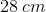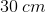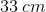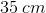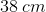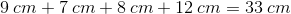# ISEE Lower Level Quantitative : How to find perimeter

## Example Questions

### Example Question #1 : Plane Geometry

Find the perimeter of the following trapezoid.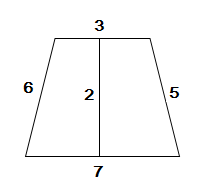Explanation:

In order to find the perimeter of the trapezoid, we have to add up all the outer sides: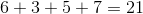### Example Question #2 : Plane Geometry

Find the perimeter of the following trapezoid.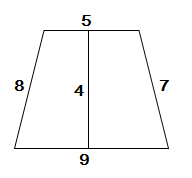Explanation:

In order to find the perimeter we must add up all the outer sides of the trapezoid.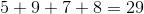### Example Question #3 : Plane Geometry

Daria found the perimeter of the below trapezoid to be 29 inches.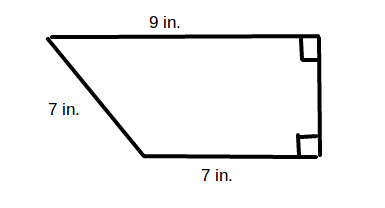What is the length of the missing side?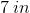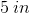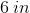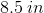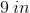Explanation:

To find the perimeter of a trapezoid, add up the lengths of its four sides. In the given trapezoid,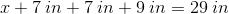To find the missing side, combine like terms and solve for: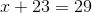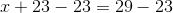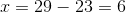The missing side of the trapezoid islong.

### Example Question #4 : Plane Geometry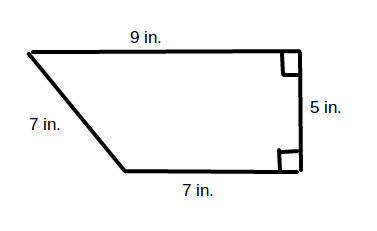What is the perimeter of the above trapezoid?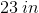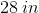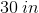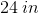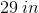Explanation:

To find the perimeter of a trapezoid, add up the lengths of the four sides. In the given trapezoid,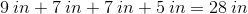### Example Question #11 : Trapezoids

Angela found the perimeter of the trapezoid below to be 35 cm.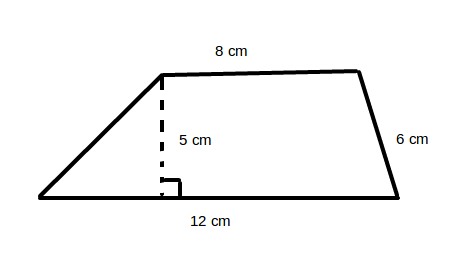What is the length of the missing side?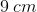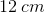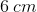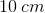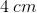Explanation:

To find the perimeter of a trapezoid, add up the lengths of the four sides. In the given trapezoid,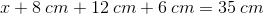To find the missing side, combine like terms and solve for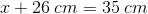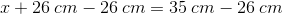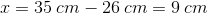The length of the missing side is thus.

### Example Question #12 : Trapezoids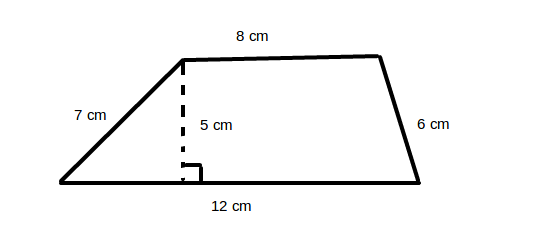What is the perimeter of the above trapezoid?